3.4 The Minimum Chi-Square Method

The method is an extension of the chi-square goodness-of-fit test described in Section 4.2. It will be seen that it is closely related to least squares and weighted least squares methods; the minimum chi-square statistic has asymptotic properties similar to ML. Pearson's (1900) paper in which it was introduced is a foundation stone of modern statistical analysis (4); a comprehensive and readable review (plus bibliography) was given by Cochran (1952).

Consider observational data which can be binned, and a model/hypothesis which predicts the population of each bin. The chi-square statistic describes the goodness-of-fit of the data to the model. If the observed numbers in each of k bins are Oi, and the expected values from the model are Ei, then this statistic is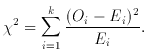(The parallel with weighted least squares is evident: the statistic is the summed squares of the residuals weighted by what is effectively the variance if the procedure is governed by Poisson statistics.) The null hypotheses H0 (see Section 4.1) is that the proportion of objects falling in each category is Ei; the chi-square procedure tests whether the Oi are sufficiently close to Ei to be likely to have occurred under H0. The sampling distribution under H0 of the statistic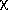2 follows the chi-square distribution (Fig. 4) with v = (k - 1) degrees of freedom. Table A III presents critical values; if2 exceeds these values, H0 is rejected at that level of significance.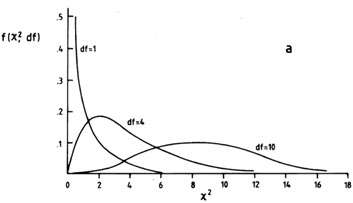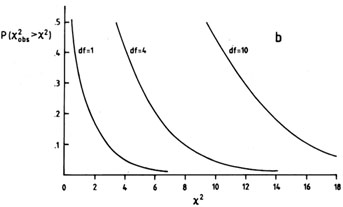Fig. 4. The2 distribution. (a) f (2, df), the probability density function of2 for df degrees of freedom. (b) The distribution function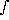2f (2, df) d2 of Table III, consulted to determine if2 is ``too large'' for a good fit, or ``large enough'' to reject H0.

The premise of the chi-square test then is that the deviations from Ei are due to statistical fluctuations from limited numbers of observations per bin, i.e. ``noise'' or Poisson statistics, and the chi-square distribution simply gives the probability that the chance deviations from Ei are as large as the observations Oi imply.

 Number of parameters Significance1 2 3 0.68 1.00 2.30 3.50 0.90 2.71 4.61 6.25 0.99 6.63 9.21 11.30

There is good news and bad news about the chi-square test. First the good: it is a test of which most scientists have heard, with which many are comfortable, and from which some are even prepared to accept the results. Moreover, because2 is additive, the results of different data sets which may fall in different bins, bin sizes, or which may apply to different aspects of the same model, may be tested all at once. The contribution to2 of each bin may be examined and regions of exceptionally good or bad fit delineated. In addition,2 is easily computed, and its significance readily estimated as follows. The mean of the chi-square distribution equals the number of degrees of freedom, while the variance equals twice the number of degrees of freedom; see plots of the function in Fig. 4. So as another rule of thumb, if2 should come out (for more than four bins) as ~ (number of bins - 1) then accept H0. But if2 exceeds twice (number of bins - 1), H0 will probably be rejected.

Now the bad news: the data must be binned to apply the test, and the bin populations must reach a certain size because it is obvious that instability results as Ei -> 0. As another rule of thumb then: > 80 per cent of the bins must have Ei > 5. Bins may have to be combined to ensure this, an operation that is perfectly permissible for the test. However, the binning of data in general, and certainly the combining of bins, results in loss of efficiency and information, resolution in particular.

The minimum chi-square method of model-fitting consists of minimizing the2 statistic by varying the parameters of the model. The premise on which this technique is based is obvious from the foregoing - the model is assumed to be qualitatively correct, and is adjusted to minimize (via2) the differences between the Ei and Oi which are deemed to be due solely to statistical fluctuations. In practice, the parameter search is easy enough (with computers) as long as the number of parameters is less than four; if four or more, then sophisticated search procedures may be necessary. The appropriate number of degrees of freedom to associate with2 is [k - 1 - (number of parameters)].

The essential question, having found appropriate parameters, is to estimate confidence limits for them. The answer is as given by Avni (1976): the region of confidence (significance level) is defined by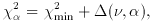whereis from Table 1.

[It is interesting to note that (a) thedepends only on the number of parameters involved, and not on the goodness-of-fit (2min) actually achieved, and (b) there is an alternative answer given by Cline & Lesser (1970) which must be in error: the result obtained by Avni has been tested with Monte Carlo experiments by Avni himself and by M. Birkinshaw (personal communication).]

By way of example, see Fig. 5. The model to describe the distribution (Fig. 5a) requires two parameters,and k. Contours of2 resulting from the parameter search are shown in Fig. 5(b). When the Avni prescription is applied, it gives20.68 =2min + 2.30, for the value corresponding to 1(significance level = 0.68); the contour20.68 = 6.2 defines a region of confidence in the (, k) plane corresponding to the 1level of significance. (Because the range of interest forwas limited from other considerations to 1.9 << 2.4, the parameter search was not extended to define this contour fully.) A cut along a line of constantis shown in Fig. 5(c); the calculation of2 defines upper and lower values of k corresponding to= 1 for this particular.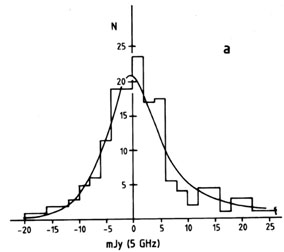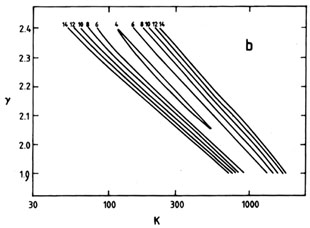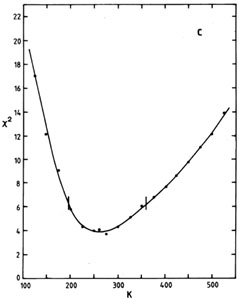Fig. 5. An example of model-fitting via the minimum-2 technique. The object of the experiment was to obtain a series of radio background-deflection measurements from which to determine parameters describing the number count [N (S) relation] of faint extragalactic sources at 5 GHz. A power-law form N = kSwas assumed, the parametersand K to be determined. (a) Binned observations of the background deflections measured. The smooth curve shows the optimum model found from the minimum-2 procedure. (b) Contours of2 in the (, K) plane for the data of (a). (c) A cut through the (, K) plane at constant= 2.2 showing the ± 68.3 per cent or 1confidence limits.

A last comment on the method of minimum chi-square. The procedure has its limitations - loss of information due to binning and inapplicability to small samples. However, it has one great advantage over other model-fitting methods - the test of the optimum model is carried out for free, as part of the procedure. For instance, the example of Fig. 5 - there are seven bins, two parameters and the appropriate number of degrees of freedom is therefore four. The value of2min is about 4, just as one would have hoped, and the optimum model is thus a satisfactory fit.

4 Pearson's paper is entitled On the criterion that a given system of deviations from probable in the case of a correlated system of variables is such that it can be reasonably supposed to have arisen from random sampling. It is wonderful polemic and gives several examples of the previous abuse of statistics, covering the frequency of buttercup petals to the incompetence of Astronomers Royal. (``Perhaps the greatest defaulter in this respect is the late Sir George Biddell Airy . . . .'') He demonstrates, for extra measure, that a run of bad luck at his roulette wheel, Monte Carlo, in 1892 July had 1 chance in 1029 of arising by chance; he avoids any libel by phrasing his conclusion ``. . . . it will be more than ever evident how little chance had to do with the results . . .''. Back.